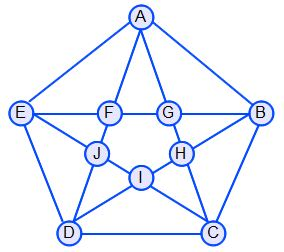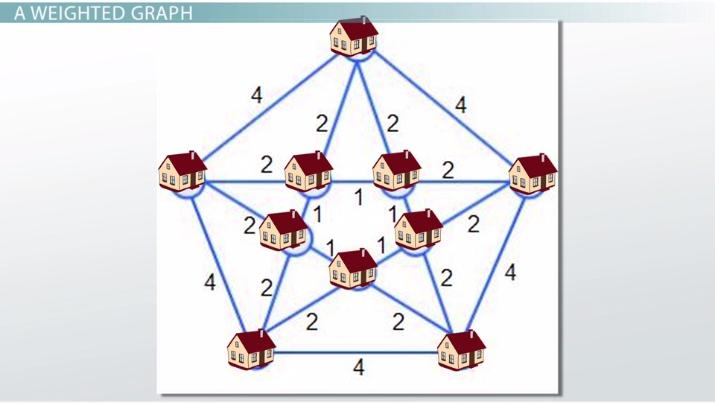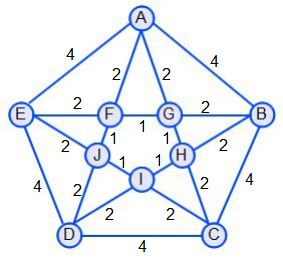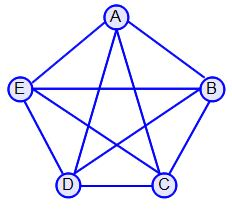Assessing Weighted & Complete Graphs for Hamilton Circuits

Lesson Transcript
Instructor: Yuanxin (Amy) Yang Alcocer

Amy has a master's degree in secondary education and has been teaching math for over 9 years. Amy has worked with students at all levels from those with special needs to those that are gifted.

A Hamilton circuit is a route found on a graph that touches each point once and returns to the starting point. Explore the properties of a Hamilton circuit, learn what a weighted graph is, examine a complete graph, and consider an example. Updated: 11/01/2021

A Hamilton Circuit

Imagine that you're a salesman. You want to visit each house in a certain neighborhood. You have drawn a map of the neighborhood in graph form using dots for houses and lines for the roads connecting the houses. You want to find a route that takes you to each house just once and takes you back to where you started. This type of route is called a Hamilton circuit in graph theory. Such a route visits each vertex just once, and you end where you start. Let's take a look at the graph you have drawn for the neighborhood:One Hamilton circuit that you can take is with you starting at point B, for example. Then going to points C, D, E, A, G, F, J, I, H and then back to B where you started. You have visited each point or house exactly once, and you ended up where you began.An error occurred trying to load this video.

Try refreshing the page, or contact customer support.

Coming up next: The Traveling Salesman Problem in Computation

You're on a roll. Keep up the good work!

Replay
Your next lesson will play in 10 seconds
• 0:01 A Hamilton Circuit
• 0:55 A Weighted Graph
• 2:38 A Complete Graph
• 3:42 Example
• 4:24 Lesson Summary
Save Save

Want to watch this again later?

Timeline
Autoplay
Autoplay
Speed Speed

A Weighted Graph

Now, what if each road had a cost associated with it? For example, say you were driving from house to house. Each road will have a different cost since some roads are longer than others and will require more gas. More gas equals more money. In graph theory, we call a graph where each edge has an associated cost or weight a weighted graph. If we added the cost for the edges, our graph might look like this now:Now, because we have a cost associated with each edge, our job of finding a Hamilton circuit gets a little bit more complicated. What we need to do now is to find a route that will cost us the least. Hey, as a salesman, you don't want to spend any more money than you have to. The cheaper it is for you to travel, the better. Now, we go along our path, we need to add up the cost associated with each edge, with each road we take. The best Hamilton circuit now is the Hamilton circuit with the least total cost. Looking at our graph and looking at how much it costs to take each road, the best Hamilton circuit is the one that zigzags between the houses. For example, if we start at house B, then go to houses H, C, I, D, J, E, F, A, G and then back to B again, our total cost is 20. Going this way is the cheapest. Of course, we could also start at house C or any other house for that matter and follow the same zigzag pattern. Any of these circuits will be the cheapest and the best. Try going other ways, and add up your costs to see if you can beat the total cost of 20.

A Complete Graph

Let's switch gears for just a moment and talk briefly about another type of graph that has a relation to the number of Hamilton circuits. This type of graph is called a complete graph. It is where each vertex is connected to every other vertex by an edge. For example, the graph below is a complete graph because you can get to any other vertex from any vertex in the graph. You don't have to pass a vertex to get to another.To unlock this lesson you must be a Study.com Member.

Register to view this lesson

Are you a student or a teacher?